## Section16.2Elasticity and Stress

### Subsection16.2.1Elasticity

Real materials react to external forces, as we have seen in the development of the Normal force. If the deformation is small compared to the physical dimensions of the object, many materials often recover the original shape when the external force is removed. This property is called elasticity.

You can see the elastic behavior clearly when you hang weights by a wire as shown in Figure 16.2.1. The reaction force here is the tension in the wire caused by the weight. Initially, the stretch and tension are linearly related, $F = k \Delta L\text{,}$ much like a spring. This part is the linear elastic region. If the tension increases beyond the linear limit, we get nonlinear behavior behavir, $F \ne k \Delta L\text{,}$ but if you remove the tension, the wire still returns to the original length.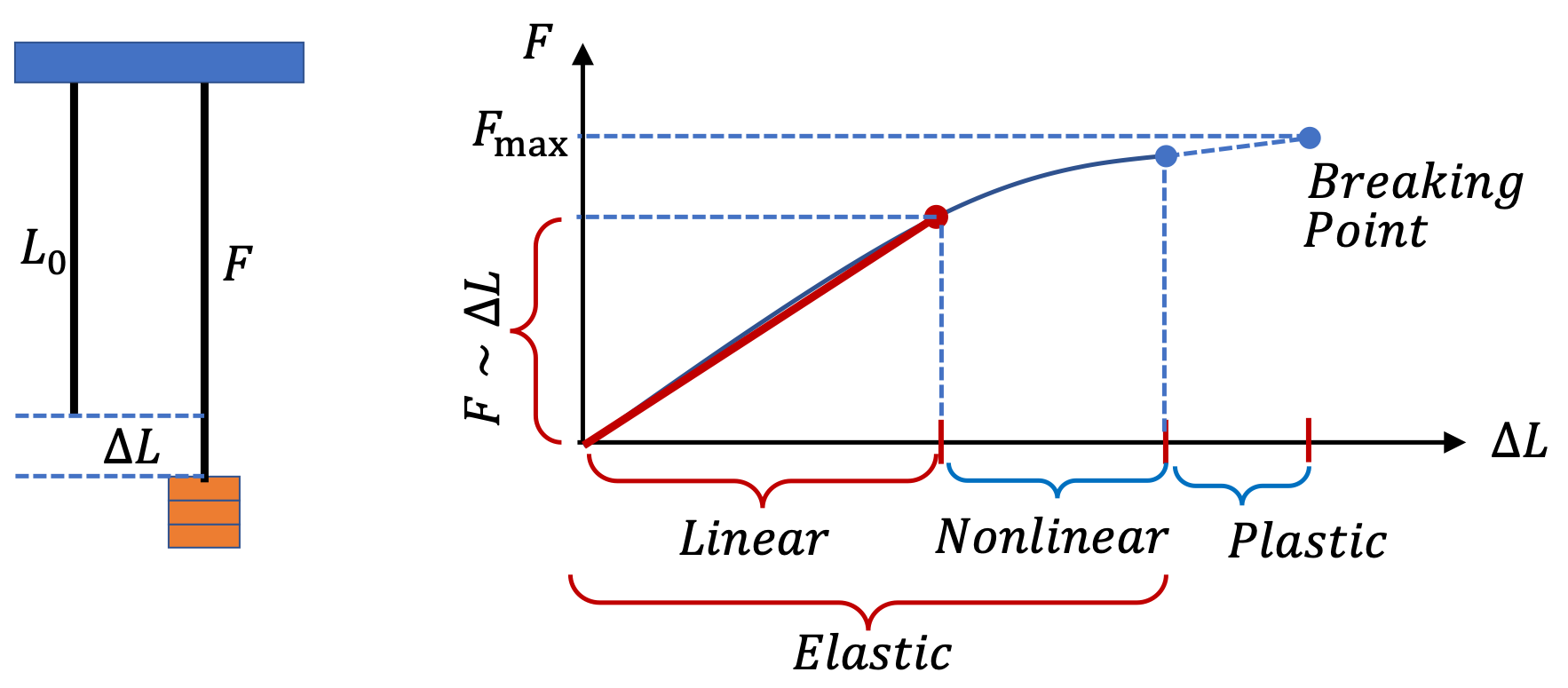Figure 16.2.1. Elasticity of a wire. The wire stretches as weights are added. Initially, tension in the wire and the stretch are linearly related, e.g., as for a spring force, $F \propto \Delta L\text{.}$ After some amount of stretch, the relation is not linear anymore, but the sire is still able to return to the original length, when we remove the weight. But if we continue to add more weights, we enter the region shown dashed, where the wire does not go back to the original length. The dashed region is the plastic region. If we continue to add more weights, eventiually, we reach a maximum tension, shown as $F_\text{max}\text{,}$ at which point, the wire breaks.

Beyond the elastic limit, there is a region, where wire still stretches, but when you remove the tension-causing weights, the wire is pemanently elongated. This is called plastic region.

If you continue to add more weight to the wire, eventually the wire will break at some point, called the breaking point. The breaking point gives us information about the ultimate strength of the material.

We will be concerned with the elastic range here since we want a predictable linear restoring force to help analyze the equilibrium behavior of structures so as to prevent permanent deformation or fracture.

In the linear elastic regime, the elongation $\Delta L$ is proportional to the force $F$ applied to the wire. Not only that, but longer wires stretch more, i.e., $\Delta L$ is proportional to the length $L$ of the wire.

\begin{equation*} \Delta L \propto F \times L. \end{equation*}

We can rewrite this as

\begin{equation} F = \alpha \dfrac{\Delta L}{L},\label{eq-hooke-law-int-1}\tag{16.2.1} \end{equation}

where $\alpha$ is a constant. By analogy with spring, we can identify $\alpha/L$ as “spring constant” of the wire.

This observation was first made by Robert Hooke (1635-1703), a contemporary of Isaac Newton, and is often called Hooke's law, which states that “Ut tensio sic uis,” in Latin, meaning “As the extension, so the force”.

The reason for a material “to act like” springs can be traced to the inter-atomic bonds in materials as illustrated in Figure 16.2.2, which have a spring-like behavior for small displacement from equilibrium.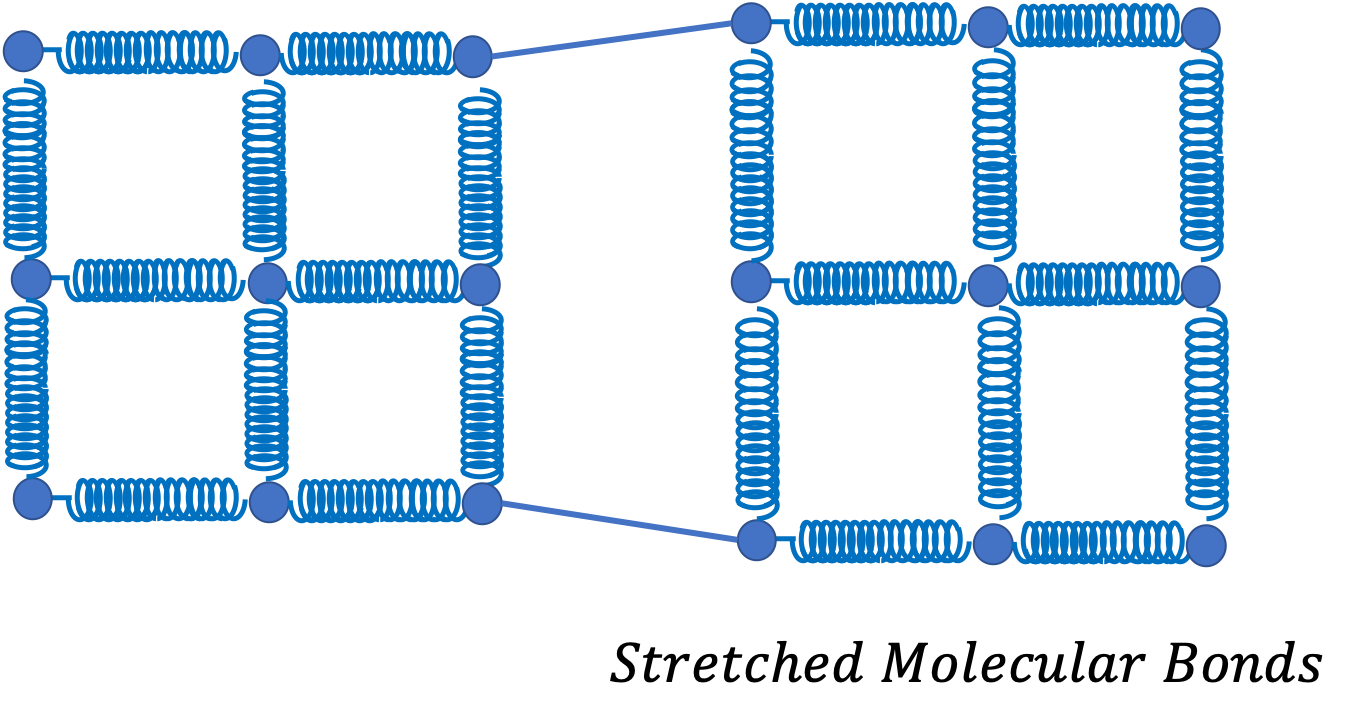Figure 16.2.2. The elastic properties of materials emerge from spring-like bonding of molecules inside the material as illustrated here. When a wire stretches vertically, the “bonds” stretch in that direction, creating a restoring force that pulls on both ends. These molecular forces are responsible for stress in the material.

### Subsection16.2.2Strain

From Eq. (16.2.1), we note that force is proportional to the fractional change of the wire. We call the fractional change in length the strain or relative deformation of length. We will denote strain by the Greek letter epsilon, $\epsilon\text{.}$

\begin{equation} \text{Strain} = \dfrac{\text{Deformation}}{\text{Original Length}}.\tag{16.2.2} \end{equation}

that is,

\begin{equation} \epsilon = \dfrac{\Delta L}{L}.\label{eq-strain-definition}\tag{16.2.3} \end{equation}

Clearly, strain is a dimensionless quantity since it is a ratio of two lengths.

There are other types of strain when we have deformation of the area or volume. The relative change in volume is called bulk strain, which we can denote by $\epsilon_V\text{.}$

\begin{equation} \epsilon_V = \dfrac{\Delta V}{V},\label{eq-bulk-strain-definition}\tag{16.2.4} \end{equation}

where $V$ is volume and $\Delta V$ the change in volume. We will discuss other types of strain in another section.

### Subsection16.2.3Stress

When you hang different masses to a wire the strain in the wire and the force exerted on the wire are linearly related, as seen above by plotting $F$ vs $\epsilon=\Delta L/L\text{.}$

When you perform the same same experiment on wires of different thicknesses, you get different plots. However, when you plot $F/A$ vs $\epsilon=\Delta L/L\text{,}$ wires of all thicknesses of the same material, fall in one line as shown schematically in Figure 16.2.3. That means $F/A$ captures more universal physics.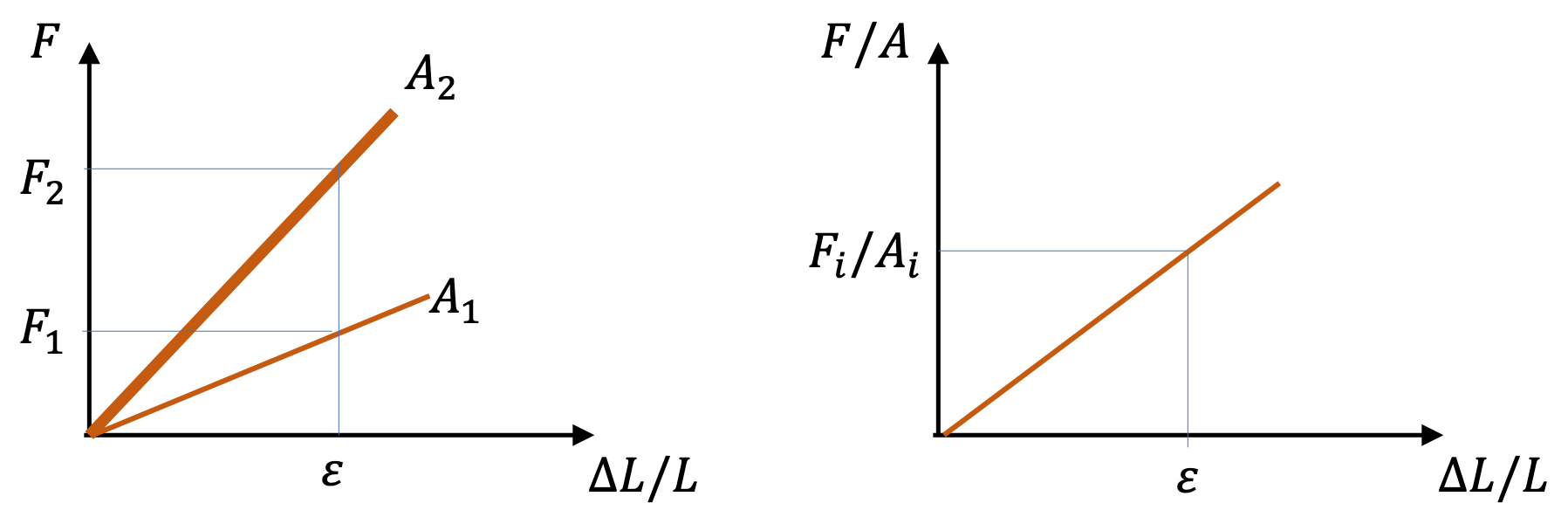Figure 16.2.3. The two plots demostrate that the effectiveness of a force to cause a strain in a material is best captured by the stress and not the force alone. On the left are plots of force versus strain of wires of two different areas of cross-section. When you plot the stress in each wire versus strain, points for same strain from the two wires $(\epsilon, F_1)$ and $(\epsilon, F_2)$ map to the same point $(\epsilon, F_i/A_i = F_1/A_1=F_2/A_2)\text{.}$

Force per unit area is called stress. We will denote stress by the Greek symbol sigma, $\sigma\text{.}$

\begin{equation} \text{Stress} = \dfrac{\text{Magnitude of Force}}{\text{Area of cross-section}},\label{eq-stress-definition}\tag{16.2.5} \end{equation}

that is,

\begin{equation} \sigma = \dfrac{F}{A}.\tag{16.2.6} \end{equation}

Note that stress will have direction since force has direction. Even the area of cross-section has an orientation. For instance, the six sides of a cube have different orientations in space. Therefore, in general, the direction of stress is dependent on the direction of the force and the orientation of the area upon which the force acts.

The dimension of stress will come from the ratio of the dimensions of force and area.

\begin{equation} [\sigma] = \dfrac{[F]}{[A]} = \dfrac{[M][L]/[T]^2}{[L]^2} = \dfrac{[M]}{[L][T]^2}.\tag{16.2.7} \end{equation}

This gives the unit of stress to be $\text{N/m}^2\text{,}$ which is also called Pascal denoted by $\text{Pa}\text{.}$

A heavy machine, of mass $2,000\text{ kg}\text{,}$ is placed on a table of mass $100\text{ kg}\text{.}$ The surface area of contact between the machine and table is $1000\text{ cm}^2\text{.}$ The table has four legs, each with the area of contact with the floor equal to $20\text{ cm}^2\text{.}$

(a) What is the stress in the table in the region where the machine is placed?

(b) What is the stress in the legs of the table?Hint

(a) Stress depends on the area also.

(a) $1.96\times 10^{5}\text{ N/m}^2\text{,}$ (b) $2.45\times 10^{6}\text{ N/m}^2\text{.}$

Solution 1 (a)

(a) The machine will have to be supported by the compression of the molecular bonds in the table below where the machine is placed. The balancing of the weight of the machine gives us the force,

\begin{equation*} F = 2000\times 9.81 = 19620\text{ N}. \end{equation*}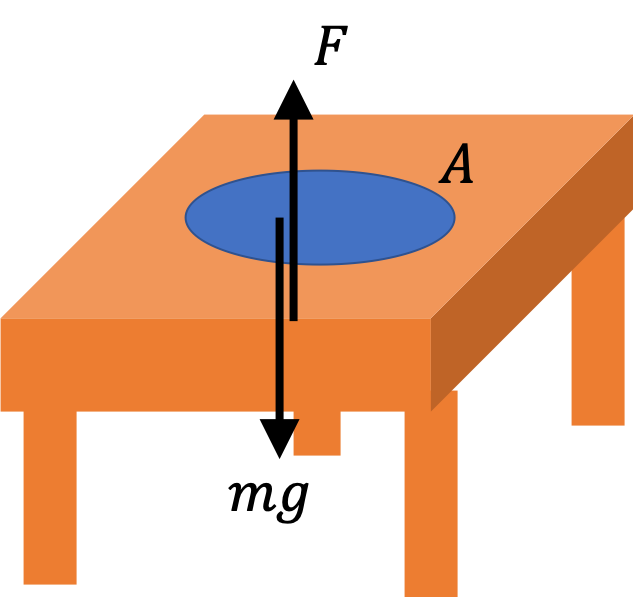This force acts over the area

\begin{equation*} A = 1000\times 10^{-4} \text{ m}^2. \end{equation*}

Therefore, stress will be

\begin{equation*} \dfrac{F}{A} = \dfrac{19620}{1000\times 10^{-4} } = 1.96\times 10^{5}\text{ N/m}^2. \end{equation*}
Solution 2 (b)

(b) The weight of the machine is now balanced by four normal forces, one on each leg, from the floor. The molecular forces in the legs of the table must balance these normal forces. Let $F$ be force in each leg.

\begin{equation*} F = \dfrac{2000\times 9.81}{4} = 4,910\text{ N}. \end{equation*}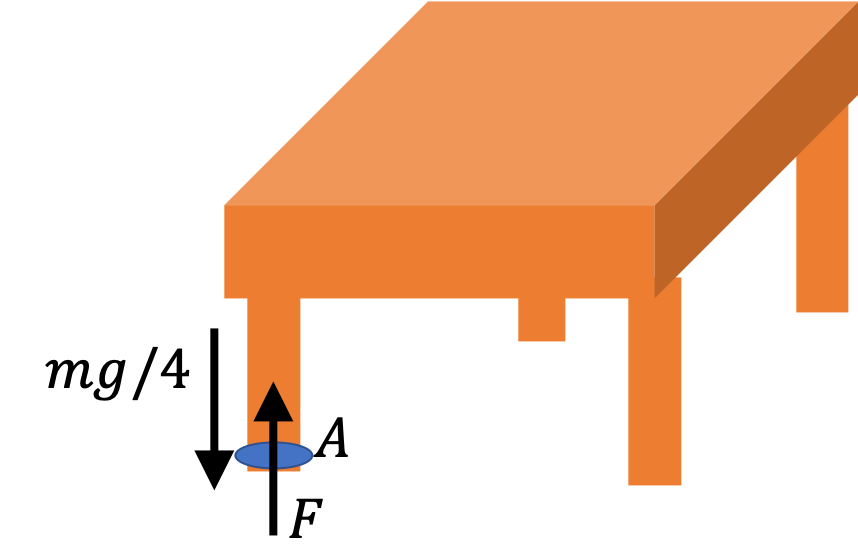This acts over the area of contact

\begin{equation*} A = 20\text{ cm}^2 = 2.0\times 10^{-3}\text{ m}^2. \end{equation*}

Therefore, stress in the legs is

\begin{equation*} \dfrac{F}{A} = \dfrac{4,910\text{ N}}{2.0\times 10^{-3}\text{ m}^2} = 2.45\times 10^{6}\text{ N/m}^2. \end{equation*}

### Subsection16.2.4General Hooke's Law

The right side part of Figure 16.2.3 says that stress in the material is directly proportional to its strain. This observation is called Hooke's law, named after Robert Hooke (1635 - 1703).

\begin{equation} \sigma = Y \, \epsilon.\label{eq-hooke-law-general}\tag{16.2.8} \end{equation}

The proportionality constant $Y$ is called Young's modulus. Young's modulus is a characteristic of the material, independent of the geometrical aspects of a sample.

The unit of Young's modulus is the same as that of stress, i.e., $\text{N/m}^2$ or Pascal, since strain in unitless.

A more flexible material has smaller $Y\text{.}$ From wikipedia, $Y_\text{rubber} = 0.01\text{-}0.1\text{ GPa}$ and that of diamond is $Y_\text{diamond} = 1,050\text{-}1,210\text{ GPa}\text{.}$ Here $G$ stands for Giga, i.e., a factor of $10^9\text{.}$

When we hang a 30-kg block from a 1-mm diameter steel wire, the wire stretches. The Young's modulus of steel is $Y_\text{steel}=215.3\text{ GPa}\text{.}$ Find (a) the stress in the wire and (b) the percent elongation.

Hint

(a) Use definition, (b) Make sure to convert units.

(a) $3.75\times 10^{8}\text{ Pa}\text{,}$ (b) $0.174\%\text{.}$

Solution

(a) The area of cross-section over which weight acts is

\begin{equation*} A = \dfrac{\pi D^2}{4} = 7.85 \times 10^{-7}\text{ m}^2. \end{equation*}

The force is

\begin{equation*} F = mg = 30\times 9.81 = 294.3\text{ N}. \end{equation*}

Therefore, the stress in the wire is

\begin{equation*} \text{Stress } = \dfrac{294.3}{ 7.85 \times 10^{-7}} = 3.75\times 10^{8}\text{ Pa}. \end{equation*}

The Young's Modulus

\begin{equation*} Y = 215.3\text{ GPa} = 215.3\times 10^9 = 2.153\times 10^{11}\text{ Pa}. \end{equation*}

Dividing the stress in the wire by $Y$ will give us the strain.

\begin{equation*} \dfrac{\Delta L}{L} = \dfrac{\text{Stress}}{Y} = \dfrac{3.75\times 10^{8}}{2.153\times 10^{11}} = 0.00174. \end{equation*}

Multiplying this by 100, we get the percent elongation, which is $0.174\%\text{.}$

How much mass must be hung from a 2-mm diameter steel wire before it breaks?

Data: Breaking Stress of steel = $400\text{ MPa}\text{.}$

Hint

Set Stress to breaking stress and solve for $M\text{.}$

$128\text{ kg} \text{.}$

Solution

Let $M$ be the mass that will break the steel wire. Let us set the stress caused by the weight of this mass just before breaking to the breaking stress.

\begin{equation*} \dfrac{Mg}{A} = Y_\text{break}. \end{equation*}

Therefore,

\begin{equation*} M = Y_\text{break} A/g = 400\times 10^{6} \times \pi \times 10^{-6}/9.81 = 128\text{ kg}. \end{equation*}

Suppose a $100\text{-kg}$ person supports all her weight on one leg. Assuming the weight is supported by the long bone of the thigh (called femur) and modeling the bone as a solid cylinder of diameter $5\text{ cm}$ and length $30\text{ cm}\text{.}$ Data: Young's modulus of cortical bone = $250 \text{ MPa}\text{.}$

By how much is the length of the bone compressed?

Hint

Convert everything in SI units and then use the definitions.

$0.6\text{ mm}$

Solution

We first compile the numbers we need in the SI units.

\begin{align*} \amp F = m g = 100\times 9.81 = 981\text{ N}, \\ \amp A = \dfrac{\pi d^2}{4} = 1.96\times 10^{-3}\text{ m}^2,\\ \amp Y = 250 \text{ MPa} = 2.50\times 10^{8}\text{ Pa},\\ \amp L = 0.30\text{ m}. \end{align*}

Therefore

\begin{align*} \Delta L\amp = \dfrac{L}{Y} \dfrac{F}{A} \\ \amp = \dfrac{0.30}{2.50\times 10^{8}}\ \dfrac{981}{1.96\times 10^{-3}} = 6.0\times 10^{-4}\text{ m}. \end{align*}

The shrinkage is $0.6\text{ mm}\text{.}$

Stress in a cable will vary if tension varies. When an elevator has acceleration pointed in upward direction, the cable will have higher tension than just the weight as required by $\vec F = m\vec a\text{.}$

An elevator of mass $4,000\text{ kg}$ is supported by a $1\text{-in}$ thick steel cable of Young's modulus $150\text{ GPa}\text{.}$ Units: $1\text{-in} = 2.54\text{ cm}\text{.}$

(a) What is the stress and strain of the cable when the elevator has zero acceleration?

(b) What is the stress and strain of the cable when the elevator has acceleration pointed up with magnitude $5\text{ m/s}^2\text{?}$

(c) What is the stress and strain of the cable when the elevator has acceleration pointed down with magnitude $5\text{ m/s}^2\text{?}$

Hint

(a) Work in same system of units. (b) Find tension by $\vec F = m \vec a\text{.}$ (c) Find tension by $\vec F = m\vec a\text{.}$

(a) $7.74 \times 10^{7} \text{ Pa},\ 5.16\times 10^{-4}\text{,}$ (b) $1.17\times 10^{8} \text{ Pa},\ 7.8\times 10^{-4}\text{,}$ (c) $3.7\times 10^{7} \text{ Pa},\ 2.45\times 10^{-4}\text{.}$

Solution 1 (a)

(a) Let us firs work out quantities we will need using SI units.

\begin{align*} W \amp = m g = 4,000\times 9.81 = 39240.0\text{ N}, \\ A \amp = \pi D^2/4 = \pi\times \left( 2.54 \times 10^{-2} \right)^2/4,\\ \amp = 5.067\times 10^{-4}\text{ m}^2,\\ Y \amp = 150\text{ GPa} = 1.50\times 10^{11}\text{ Pa}. \end{align*}

Since acceleration is zero, the tension in the cable is

\begin{equation*} F = W = 39240\text{ N}. \end{equation*}

Therefore, stress in the cable is

\begin{equation*} \text{Stress } = \dfrac{F}{A} = \dfrac{39240}{ 5.067\times 10^{-4} }= 7.74 \times 10^{7} \text{ Pa}, \end{equation*}

and strain in the cable is

\begin{equation*} \dfrac{\Delta L}{L} = \dfrac{\text{Stress}}{Y} = \dfrac{7.74 \times 10^{7} }{1.50\times 10^{11} } = 5.16\times 10^{-4}. \end{equation*}
Solution 2 (b)

(b) In this part, the elevator has upward acceleration. The diagram on the right shows the forces. The equation of motion along vertical direction gives

\begin{equation*} F-mg = ma. \end{equation*}

Therefore, the tension in the cable will be

\begin{equation*} F = 39240 + 4000\times 5 = 59240\text{ N}. \end{equation*}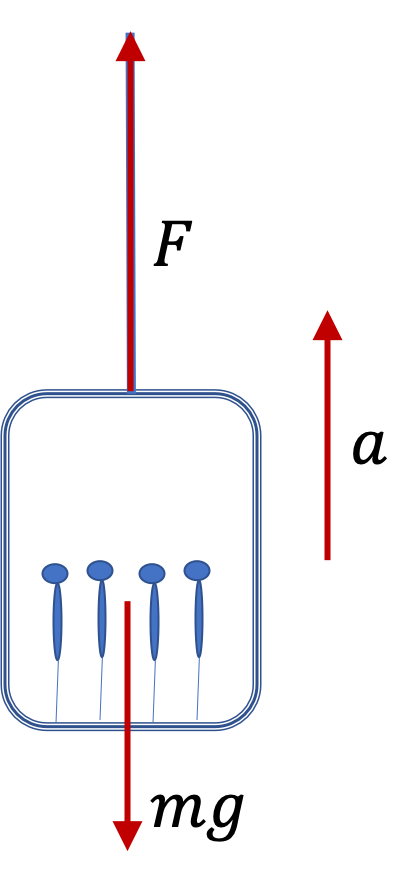Therefore, stress in the cable is

\begin{equation*} \text{Stress } = \dfrac{F}{A} = \dfrac{ 59240}{ 5.067\times 10^{-4} }= 1.17\times 10^{8} \text{ Pa}, \end{equation*}

and strain in the cable is

\begin{equation*} \dfrac{\Delta L}{L} = \dfrac{\text{Stress}}{Y} = \dfrac{1.17 \times 10^{8} }{1.50\times 10^{11} } =7.8\times 10^{-4}. \end{equation*}
Solution 3 (c)

(c) In this part, the elevator has downward acceleration. The diagram on the right shows the forces. The equation of motion along vertical direction gives

\begin{equation*} F-mg = - ma. \end{equation*}

Therefore, the tension in the cable will be

\begin{equation*} F = 39240 - 4000\times 5 = 19240\text{ N}. \end{equation*}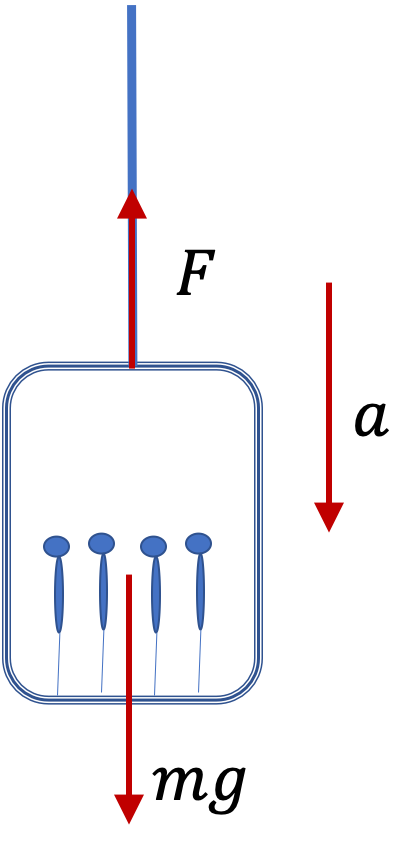Therefore, stress in the cable is

\begin{equation*} \text{Stress } = \dfrac{F}{A} = \dfrac{ 19240}{ 5.067\times 10^{-4} }= 3.7\times 10^{7} \text{ Pa}, \end{equation*}

and strain in the cable is

\begin{equation*} \dfrac{\Delta L}{L} = \dfrac{\text{Stress}}{Y} = \dfrac{3.7 \times 10^7}{1.50\times 10^{11} } = 2.45\times 10^{-4}. \end{equation*}

A beam that extends beyond its support is called a cantilever. Consider a cantilever of length $L$ and mass $M$ resting and bolted to two supports, one at the end and the other is at a distance $D$ from it as shown in Figure 16.2.10.

(a) Find the conditions on the forces such that the cantilever is in static equilibrium.

(b) For $L = 30\text{ m}\text{,}$ $M = 1000\text{ kg}\text{,}$ and $D = 12\text{ m}\text{,}$ find the magnitude and directions of the forces on the beam from the supports.

(c) The bolt provide an area of contact over which contact forces act. Suppose, the area of contact of the bolt at the support at the end be $60\text{ mm}^2$ . Find the stress in the bolt for the values given in (b).

Hint

Use the results of Checkpoint 16.1.9.

(a) Horizontal and vertical forces from the left post : $F_{11} = 0\text{,}$ $F_{12} = Mg\left(1-\frac{L}{2D} \right)\text{;}$ Vertical force from the second post $F_2 = \left(\frac{L}{2D} \right)Mg\text{.}$ (b) $0,\ -2500\text{ N},\ 12000\text{ N}\text{.}$ (c) $4.17\times 10^{7}\text{ N/m}^2\text{.}$
(a) and (b) are done in Checkpoint 16.1.9. I will just restate the answers here (a) Horizontal and vertical forces from the left post : $F_{11} = 0\text{,}$ $F_{12} = Mg\left(1-\frac{L}{2D} \right)\text{;}$ Vertical force from the second post $F_2 = \left(\frac{L}{2D} \right)Mg\text{.}$ (b) $0,\ -2500\text{ N},\ 12000\text{ N}\text{.}$